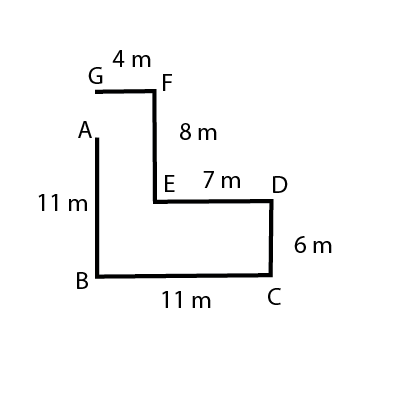# Directions Questions for Bank PO and Clerk – Online Quiz

0
948

Directions Questions for Bank Po and Clerk useful for all Banking exams like IBPS PO and IBPS Clerk and SBI exams.

Directions Sense Test for Bank Po and Clerk:

Question 1

An ant starts moving on a circle of radius 50 cm. It starts from the southern-most tip of the circumference of the circle and travels along the circumference of the circle in clockwise direction till it reaches the western-most point on the circumference of the circle. It takes a right turn and moves in a straight line for 100 cm. How far is the ant from the starting point?

Solution:

The ant’s final position is the eastern-most tip of the circumference of the circle. Since the radius of the circle is 50 cm, the distance from the starting point is $$\sqrt{50^2 + 50^2}$$ = $$50\sqrt2$$ cm

Question 2

Stephen cycled 20 km south, followed by 30 km east, and followed by 60 km north. How many km should he cycle back to reach his starting point?

Solution:

Stephen is 30 km east and 40 km north from the starting point. So, he should cycle $$\sqrt{40^2 + 30^2}$$ = 50 km to reach his starting point.

Question 3

If north-east becomes south, east becomes south-west and so on, what will north-west become?

Solution:

If north-east becomes south, north-west will become east.

Instructions

Study the given information and answer the given questions.
Point A is 11 m North of point B.
Point C is 11 m East of point B
Point D is 6 m North of point C
Point E is 7 m West of point D
Point F is 8 m North of point E
Point G is 4 m West of point F

Question 4

What is the shortest distance between point F from point A ?

Solution:$$AF^{2} = 4^{2} + 3^{2}$$

AF = 5

According to the above diagram, shortest distance between F and A will be 5 m. Hence, answer will be E

Question 5

How far and in which direction is point G from point A ?

Solution:As we can see in the above diagram that distance between G and A will be 8-(11-6) = 3 m